# # 8 指针分析-上下文敏感

## # 8.1 引入

### # 8.1.1 引例

interface Number {
int get();
}

class One implements Number {
public int get() {
return 1;
}
}

class Two implements Number {
public int get() {
return 2;
}
}

1
2
3
4
5
6
7
8
9
10
11
12
13
14
15

void main() {
Number n1, n2, x, y;
n1 = new One(); // o1
n2 = new Two(); // o2
x = id(n1);
y = id(n2);
int i = x.get();
}

Number id(Number n) {
return n;
}

1
2
3
4
5
6
7
8
9
10
11
12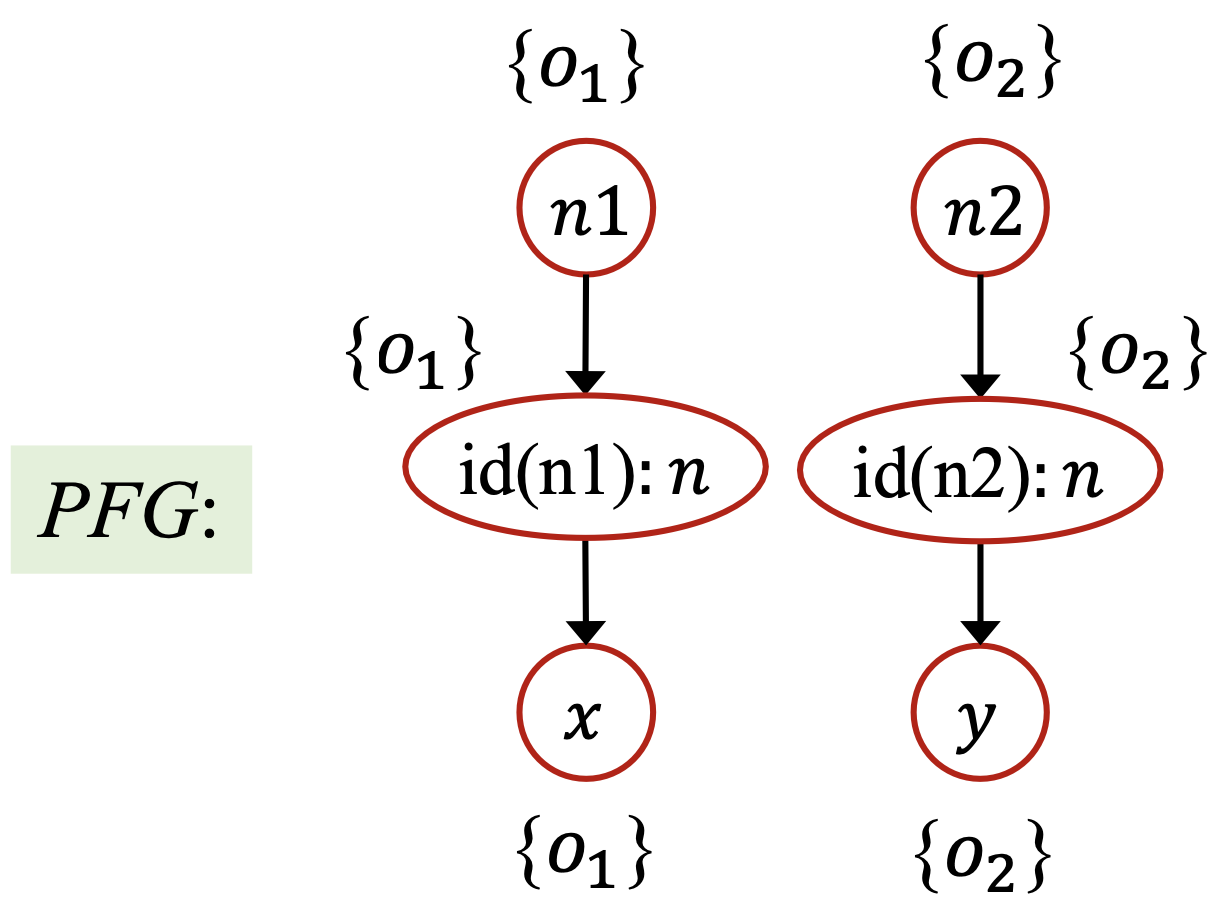### # 8.1.2 上下文不敏感与上下文敏感

• 在动态执行的过程中，每一个方法可能在不同的调用语境中被调用多次。

• 在不同的调用语境下面，方法中的变量可能会指向不同的对象。

• 在上下文不敏感的指针分析中，不同的上下文被混合在了一起，并传递给程序的其他部分（通过返回值或者副作用），这就导致了虚假的数据流。

• 比如说上面的引例，其上下文不敏感的分析结果中， $pt(x)$ 中的 $o_2$$pt(y)$ 中的 $o_1$ 都是虚假的指向对象，从而给常量传播分析造成了虚假的数据流。

#### # 基于克隆的上下文敏感

• 每个方法都被一个或者多个上下文修饰；

• 变量也被上下文修饰（继承自声明该变量的方法）；

• 对于每个上下文，都会恰有一个方法及该方法中的变量的克隆。

### # 8.1.3 上下文敏感的堆

• 抽象的对象也应当用上下文来修饰（称为堆上下文），最普遍的选择是继承该对象分配点所在方法的上下文。

• 上下文敏感的堆抽象在分配点抽象的基础上提供了一个粒度更精确的堆模型。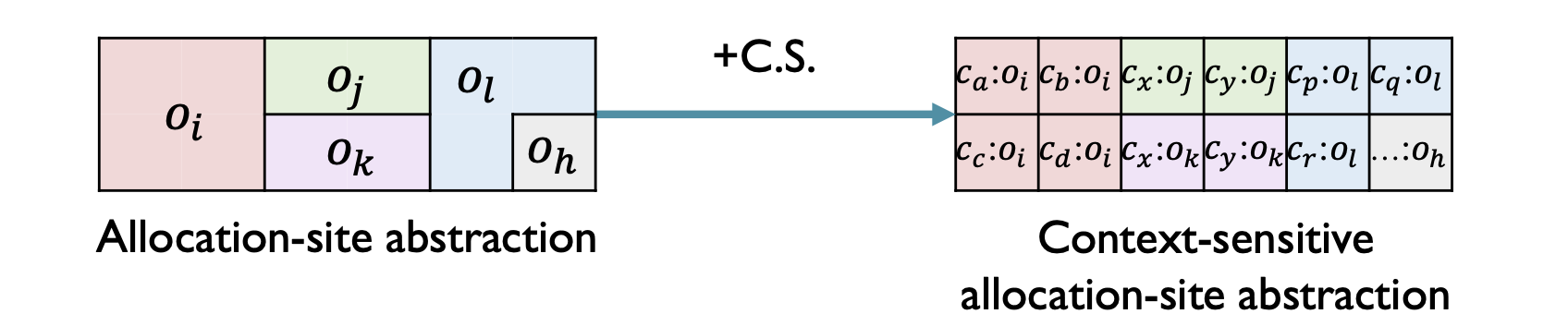• 在动态执行的过程中，一个分配点可以在不同的调用语境下创建多个对象；

• 不同的对象（有相同的分配点分配）可能会携带不同的数据流被操作，比如说在它们的字段中存储不同的值。

• 在指针分析中，不带堆上下文分析这样的代码可能会由于合并不同上下文中的数据流到一个抽象对象中而丢失精度。

• 相比之下，通过堆上下文来区分同一个分配点的不同对象能够获得不少的精度。

n1 = new One();
n2 = new Two();
x1 = newX(n1);
x2 = newX(n2);
n = x1.f;

X newX(Number p) {
X x = new X();
x.f = p;
return x;
}
class X {
Number f;
}

1
2
3
4
5
6
7
8
9
10
11
12
13
14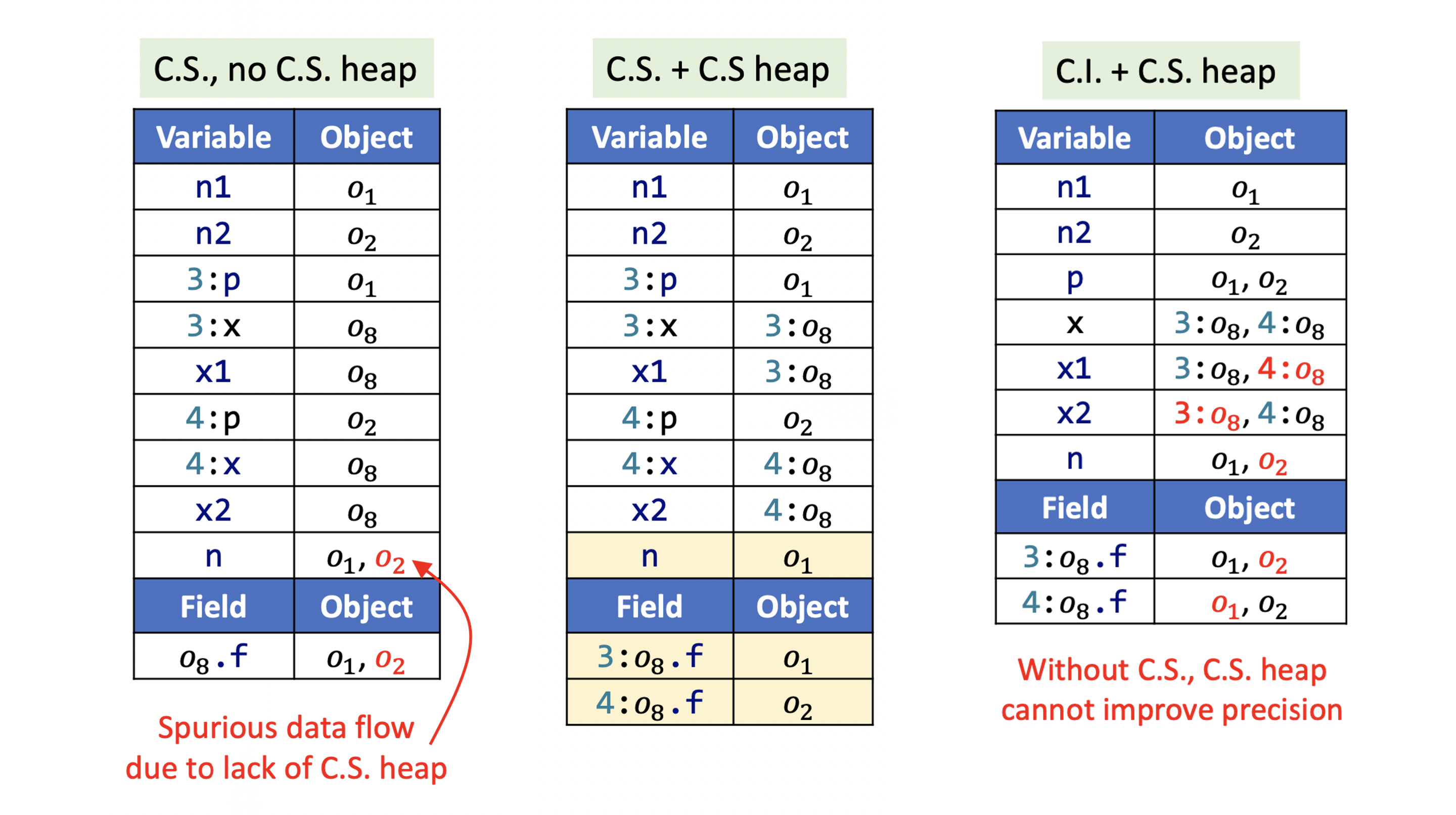## # 8.2 上下文敏感的指针分析规则

### # 8.2.2 规则

$c':o_i \in pt(c:x)$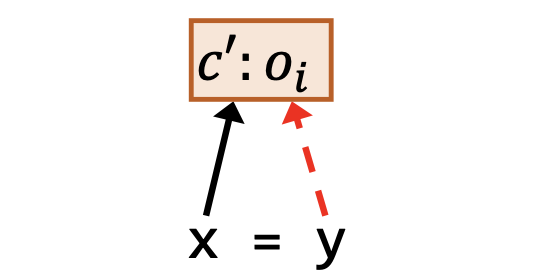$c'':o_j\in pt(c':o_i.f)$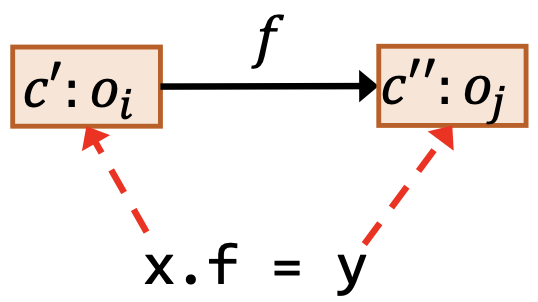$c'':o_j\in pt(c:y)$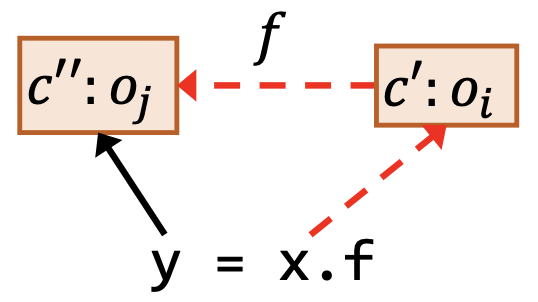$m = Dispatch(o_i, k), c^t = Select(c, l, c':o_i)$
$c'':o_u\in pt(c:a_j), 1\le j\le n$
$\underline{c''':o_v\in pt(c^t:m_{ret})}$
$c':o_i\in pt(c^t:m_{this})$
$c'':o_u\in pt(c^t:m_{p_j}), 1\le j\le n$
$c''':o_v\in pt(c:r)$

• $Dispatch(o_i, k)$ 和上一讲一致，根据 $o_i$ 的类型对虚调用 $k$ 做方法派发，从而获得一个目标方法。

• $Select(c, l, c':o_i)$ 根据在调用点 $l$ 处可获得的信息为目标方法 $m$ 选择一个上下文，即目标上下文的生成函数。

• Select 的输入是调用点 $l$ 、调用点处的上下文 $c$ 、接收对象 $c':o_i$

• Select 的输出是目标方法的的上下文 $c^{t}$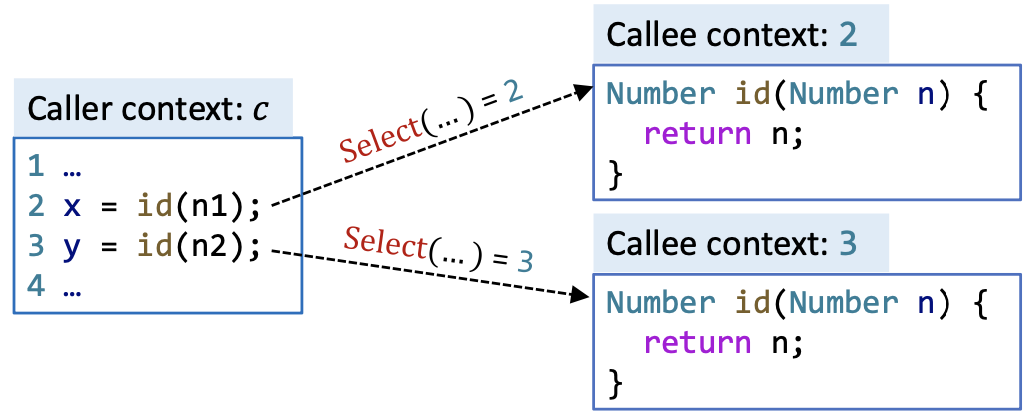## # 8.3 上下文敏感的指针分析算法

### # 8.3.1 实现思路• PFG中的结点 $n$ 代表了一个上下文敏感的变量或者一个上下文敏感的对象的某个字段，即程序中的被上下文修饰的指针。
$n \in CSPointer \subseteq (C\times V)\cup(C\times O\times F)$
• PFG中的边 $x\to y \in CSPointer \times CSPointer$ 表示指针 $x$ 指向的对象可能会流向指针 $y$ 的指向集合。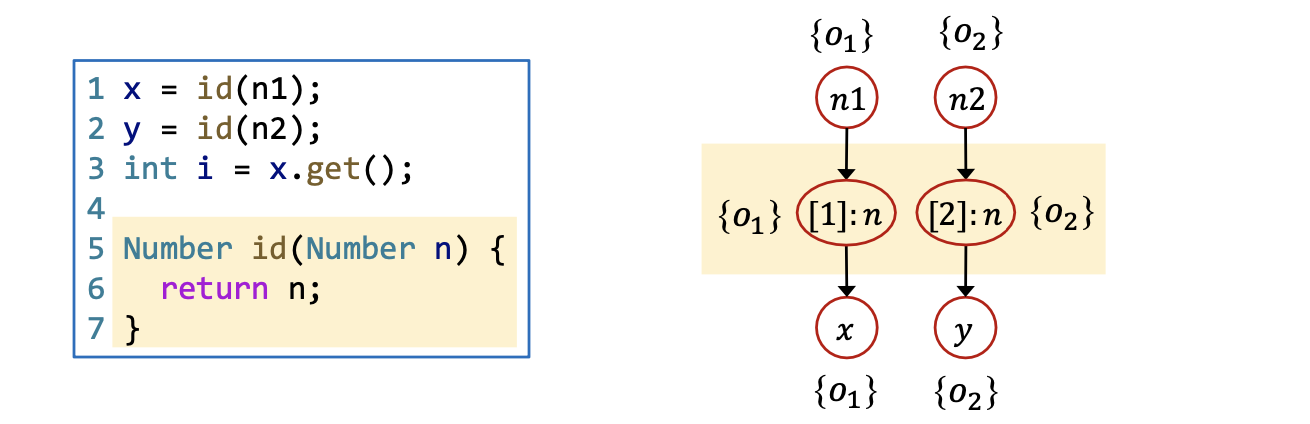$c':o_i \in pt(c:x)$
$c:y \to c:x$

$c'':o_j\in pt(c':o_i.f)$
$c:y \to c':o_i.f$

$c'':o_j\in pt(c:y)$
$c':o_i.f\to c:y$

x.k(a1, ..., an)
$c':o_i\in pt(c:x)$
$m = Dispatch(o_i, k), c^t = Select(c, l, c':o_i)$
$c'':o_u\in pt(c:a_j), 1\le j\le n$
$\underline{c''':o_v\in pt(c^t:m_{ret})}$
$c':o_i\in pt(c^t:m_{this})$
$c'':o_u\in pt(c^t:m_{p_j}), 1\le j\le n$
$c''':o_v\in pt(c:r)$
$c:a_1\to c^t:m_{p_1}$
$... ...$
$c:a_n \to c^t:m_{p_n}$
$c^t:m_{ret}\to c:r$

## # 8.4 上下文敏感性的各种变体

### # 8.4.1 关于上下文敏感性

• Select 函数的输入是调用点处的上下文 $c$ ，调用点 $l$ ，以及调用方法的带有堆上下文的接收对象 $c':o_i$

• Select 函数的输出是用于修饰目标方法上下文。

• 如果 Select 经常出现上下文重叠，指针分析的精度会受损，相应的，速度会提高；

• 如果 Select 偶尔出现上下文重叠，指针分析的精度会提高，相应的，速度会减缓。

$Select(\_, \_, \_) = []$

### # 8.4.2 调用点敏感

$c = [l', ..., l''] \Longrightarrow Select(c, l, \_) = [l', ..., l'', l]$

• 对于调用点敏感，每个上下文都由调用串的最后 $k$ 个调用点组成；

• 在实际操作中， $k$ 是一个小数字，通常不超过3；

• 方法上下文和堆上下文可能会使用不同的k。

• 比如说方法上下文的 $k = 2$ ，堆上下文的 $k = 1$ 之类的。

$c = [l_1, l_2, ..., l_k] \Longrightarrow Select(c, l, \_) = [l_2, ..., l_k, l]$

k-调用点敏感也称为 k-控制流分析（k-CFA, Control Flow Analysis） 。 具体地：

• 1-调用点敏感
$Select(\_, l, \_) = [l]$
• 2-调用点敏感
$c = [l', l''] \Longrightarrow Select(c,l,\_) = [l'', l]$

class C {
static void main() {
C c = new C();
c.m();
}

Number id(Number n) {
return n;
}
void m() {
Number n1, n2, x, y;
n1 = new One();
n2 = new Two();
x = this.id(n1);
y = this.id(n2);
x.get();
}
}

1
2
3
4
5
6
7
8
9
10
11
12
13
14
15
16
17
18### # 8.4.3 对象敏感

$c' = [o_j, ..., o_k] \Longrightarrow Select(\_, \_, c':o_i) = [o_j, ..., o_k, o_i]$

• 在方法调用的时候，使用接收对象以及接收对象的堆上下文作为被调用者的上下文（就像定义8.9中所形式化定义的那样）；

• 根据不同的对象来区分不同的数据流操作，因为流动的数据往往都是由对象携带的（至少对于OO语言来说这是很常见的）。

$c' = [o_1, ..., o_k] \Longrightarrow Select(\_, \_, c':o_i) = [o_2, ..., o_k, o_i]$

$Select(\_, \_, c':o_i) = [o_i]$

a1 = new A();
a2 = new A();
b1 = new B();
b2 = new B();
a1.set(b1);
a2.set(b2);
x = a1.get();

class A {
B f;
void set(B b) {
this.doSet(b);
}
void doSet(B p) {
this.f = p;
}
B get() {
return this.f;
}
}

1
2
3
4
5
6
7
8
9
10
11
12
13
14
15
16
17
18
19
20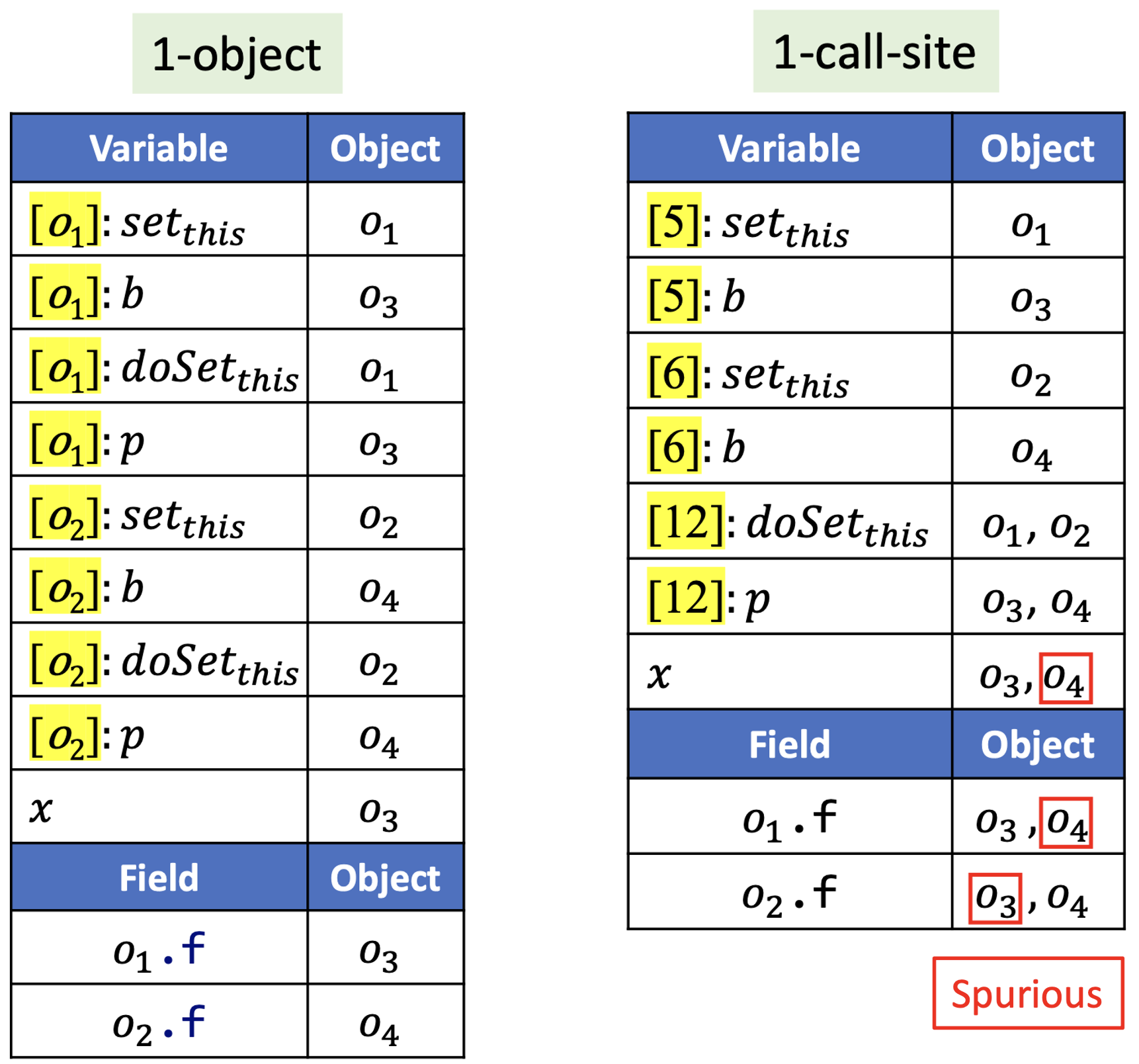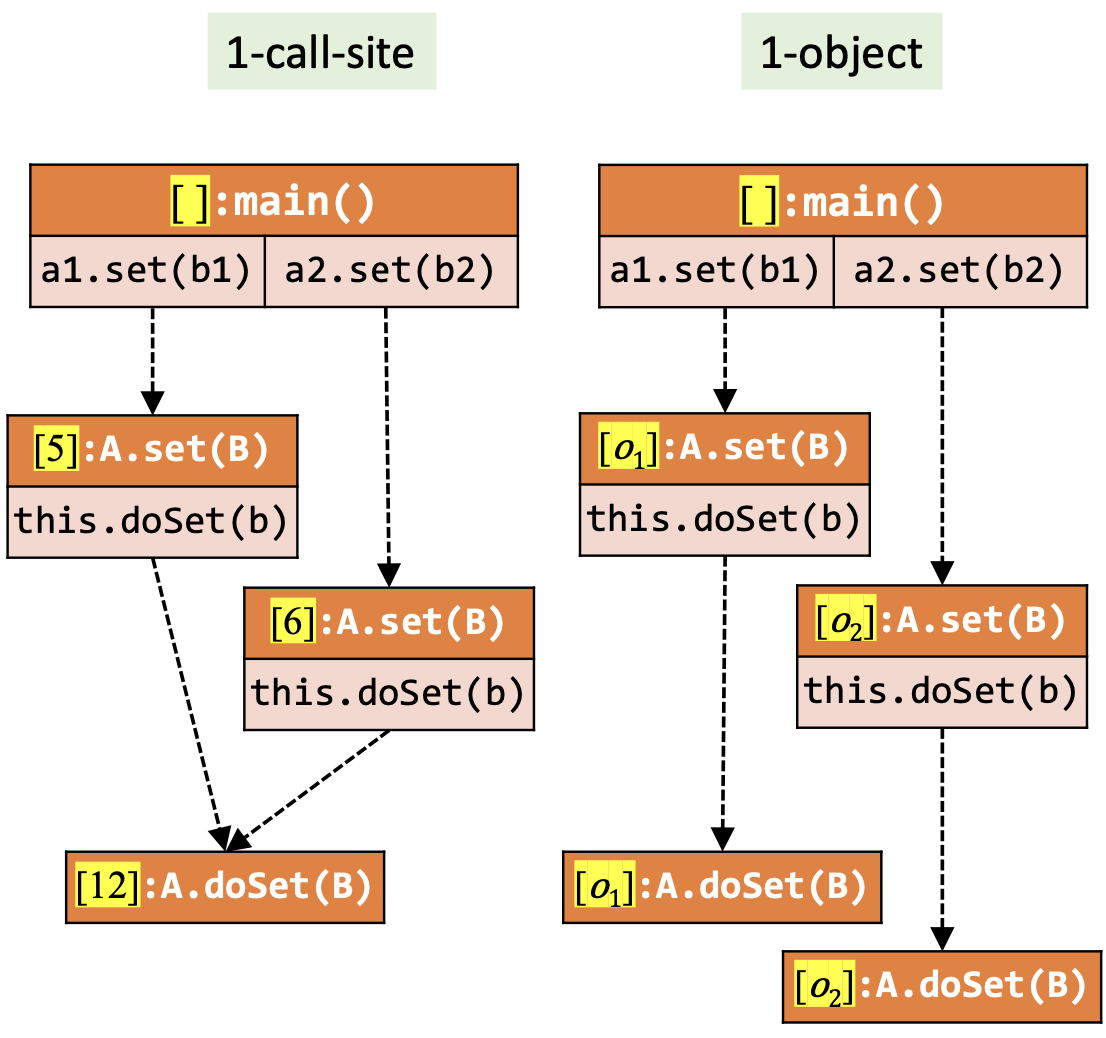### # 8.4.4 类型敏感

$c' = [t', ..., t''] \Longrightarrow Select(\underline{\ }, \underline{\ }, c':o_i) = [t', ..., t'', InType(o_i)]$

$c' = [t_1, ..., t_k] \Longrightarrow Select(\_, \_, c':o_i) = [t_2, ..., t_k, InType(o_i)]$

class X {
void main() {
Y y1 = new Y();
y1.foo();
Y y2 = new Y();
y2.foo();
Y y3 = new Y();
y3.foo();
}
}
class Y {
void foo() {}
}

1
2
3
4
5
6
7
8
9
10
11
12
13

### # 8.4.5 对比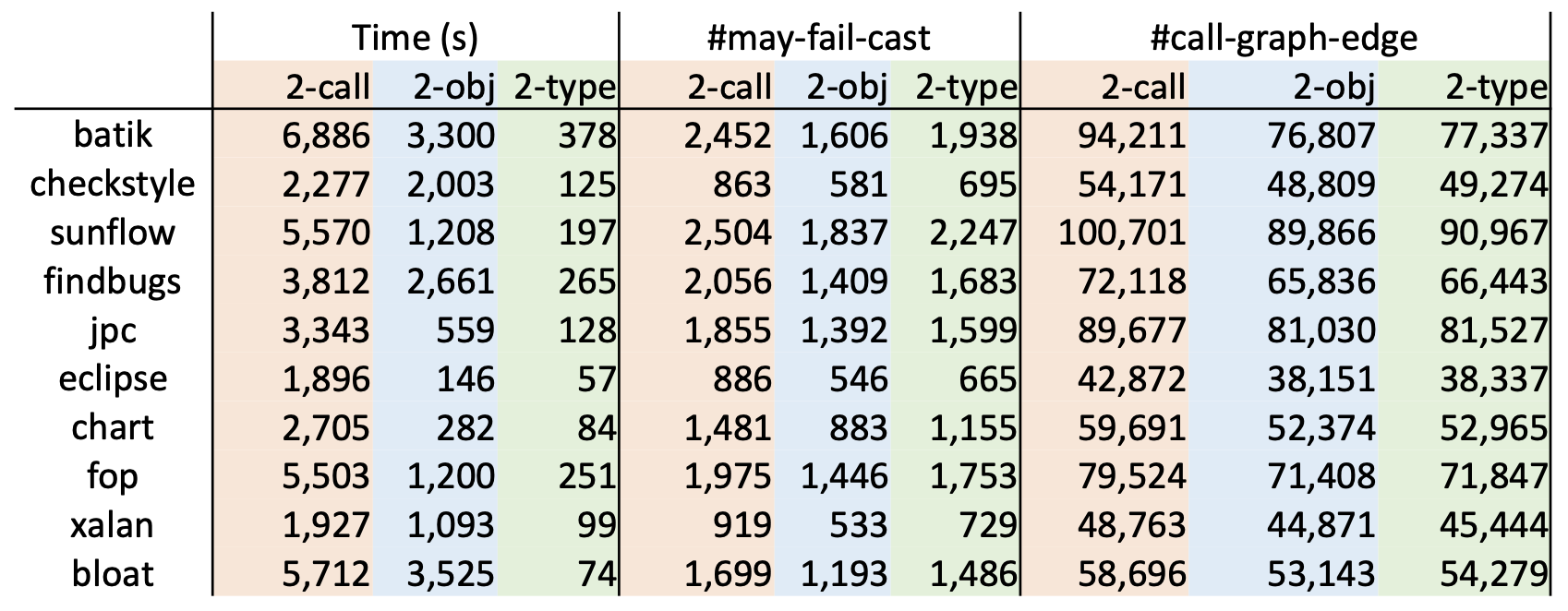• 精度方面：

• 对象敏感 > 类型敏感 > 调用点敏感
• 效率方面：

• 类型敏感 > 对象敏感 > 调用点敏感

## # 8.5 自检问题

1. 上下文敏感（Context Sensitivity, C.S.）是什么？

2. 上下文敏感堆（C.S. Heap）是什么？

3. 为什么 C.S. 和 C.S. Heap 能够提高分析精度？

4. 上下文敏感的指针分析有哪些规则？

5. 如何理解上下文敏感的指针分析算法（Algorithm for Context-sensitive Pointer Analysis）？

6. 常见的上下文敏感性变体（Context Sensitivity Variants）有哪些？

7. 常见的几种上下文变体之间的差别和联系是什么？